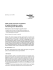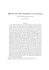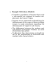# Estimation Theory Basics What is Estimation something Prior Information

## Transcription

Estimation Theory Basics What is Estimation something Prior Information
```EE 5510 Random Processes
E-1
Estimation Theory Basics
What is Estimation
Make a best determination of
something given measurements
(information) related (in a known,
or partially known way) to that
something, and possibly also given
some prior information
Prior Information may be
• a Random Variable, this is the Bayesian Approach,
• non-random, or sets
Example: Estimation of the mean of random variable:
1X
m
ˆx =
Xi
n i=1
n
is an estimator for mx = E(X), the mean of the random variable
X.
We know that m
ˆ x is a “good” estimator, since
P (|m
ˆ x − mx | < ²) → 1
by the law of large numbers.
EE 5510 Random Processes
E-2
Parameter Estimation
We are given a number of observations xi , i.e., x = [x1 , x2 , · · · , xn ]. This
sample vector of “data” is called a statistic. From this statistic we are
to estimate the desired parameter, i.e.,
θˆ = g(x)
Unbiased Estimator
An estimator is unbiased if
ˆ =θ
E(θ)
Example: The estimator m
ˆx =
1
n
Pn
i=1 xi
is an unbiased estimator since
1X
E(m
ˆ x) =
E(xi ) = mx
n i=1
n
Example: Estimation of unknown variance (and unknown mean):
1 X
σ
ˆ =
(xi − m
ˆ x )2
n − 1 i=1
n
2
This estimator is unbiased, since
!
Ã
!
Ã
n
n X
n
X
X
¡
¢
1
1
E (xi − m
xi
ˆ x )2 = E(x2i ) − 2E
xj + E
xi xj
n j=1
n2 j=1 i=1
1
n−1 2
= E(x2i ) − 2 E(x2i ) − 2
mx
n
n
n
1 X
n(n − 1) 2
+ 2
E(x2j ) +
mx
n j=1
n2
µ
¶
1
n−1 2
= E(x2i ) 1 −
−
mx
n
n
n−1
= σ2
n
EE 5510 Random Processes
E-3
Biased Coin Toss
Assume that a coin is biased and shows head (=1) with probability θ 6=
0.5, and tail with probability 1 − θ. We observe the n tosses of this coin
and are to estimate the probability θ of a head toss.
Let us form the statistic
x1 + x2 + · · · + xn
z=
n
The mean of this estimator is
1X
E(Z) =
E(Xi ) = θ
n i=1
n
and hence this estimator is unbiased.
The variance of this estimator is
¢
¡
σz2 = E (Z − θ)2
Ã
!
Ã n
!
n
n X
X
X
1
1
= E
−
2E
X
X
Xi θ + θ2
i
j
2
n i=1 j=1
n i=1
!
Ã
n
n
1 XX
= E
Xi Xj − θ2
2
n i=1 j=1
=
n−1
θ(1 − θ)
(n(n − 1)θ2 − nθ) − θ2 =
n
n
The question arrises whether this is the “best” estimator?
Minimum Variance Estimators (MVE)
Let us find the estimator which minimizes
³
ˆ2
E (θ − θ)
´
However the MVE cannot, in general, be determined, since
³
´
³
´
2
2
ˆ
ˆ
ˆ
ˆ
E (θ − θ)
= E (θ − E(θ) − (θ − E(θ))
ˆ
ˆ 2 + var(θ)
= (θ − E(θ))
ˆ 2 , called the bias, depends on the unknown
since the term (θ − E(θ))
quantity θ.
EE 5510 Random Processes
E-4
Cram´
er-Rao Lower Bound
Assumptions
We assume that we have knowledge of the parametric distribution f (x, θ),
and that this distribution is differentiable with respect to θ. We also assume that the domain of the statistic x does not depend on the parameter
θ.
Derivation
We now concentrate on unbiased estimators:
Z
E(θˆ − θ) = (θˆ − θ)f (x, θ)dx = 0
ˆ Now
due to the unbiased nature of θ.
Z
Z
d
d ˆ
(θˆ − θ)f (x, θ)dx =
(θ − θ)f (x, θ)dx
dθ
dθ
Z
Z
d
= − f (x, θ)dx + (θˆ − θ) f (x, θ)dx = 0
dθ
Using the identity
d ln(f (x, θ))
d
f (x, θ) =
f (x, θ)
dθ
dθ
we develop:
Z
d ln(f (x, θ))
f (x, θ)dx
dθ
¶2
Z µ
Z
d
ln(f
(x,
θ))
f (x, θ)dx
≤
(θˆ − θ)2 f (x, θ)dx
dθ
1 =
(θˆ − θ)
from which we find the Cram´
er-Rao lower bound (CRLB):
ˆ ≥
var(θ)
³
E
1
d ln(f (x,θ))
dθ
´2
EE 5510 Random Processes
E-5
Equality in the CRLB is achieved if equality is achieved in the Schwarz
inequality (see Page ??) used to prove it, i.e., if
d ln(f (x, θ))
= I(θ)(θˆ − θ)
dθ
¶2
d ln(f (x, θ))
I(θ) =
f (x, θ)dx
dθ
Z 2
d ln(f (x, θ))
= −
f (x, θ)dx
dθ2
Z µ
is the Fisher Information of θ contained in the observation x.
Consider n observations of a constant A in Gaussian noise, i.e.,
xi = A + ni ;
1≤i≤n
The PDF of the observation vector x = (x1 , · · · , xn ) is given by
!
Ã
n
1 X
1
exp − 2
(xi − A)2
f (x, A) =
2
(n/2)
2σ i=1
(2πσ )
We calculate
d ln(f (x, A))
1 X
n
= 2
(xi − A) = 2
dA
σ i=1
σ
n
Ã
!
1X
xi − A
n i=1
n
from which we can identify the optimal unbiased estimator as
1X
ˆ
A=
xi
n i=1
n
Furthermore
d2 ln(f (x, A))
n
=
−
= −I(A)
dA2
σ2
and the variance of the estimator is given by
ˆ =
var(A)
1
σ2
=
I(A)
n
Example:
EE 5510 Random Processes
E-6
Signals in Gaussian Noise
As an example, consider the application of a radar range estimator:
H(f )
x(t)
We transmit s(t) and receive r(t) = s(t − τ ) + nw (t), and wish to estimate
the delay τ .
We first treat the more general problem of a parameterized signal in
Gaussian noise:
xi = s[n; θ] + ni ;
1≤i≤n
We calculate
d ln(f (x, θ))
1 X
ds[n; θ]
= 2
(xi − s[n; θ])
dθ
σ i=1
dθ
n
and the second derivative
Ã
µ
¶2 !
n
2
X
d ln(f (x, θ))
ds[n; θ]
1
d s[n; θ]
=− 2
−
(xi − s[n; θ])
2
2
dθ
σ i=1
dθ
dθ
2
EE 5510 Random Processes
E-7
The Fisher information is now calculated as
µ 2
¶2
¶
n µ
d ln(f (x, θ))
1 X ds[n; θ]
I(θ) = E
=− 2
dθ2
σ i=1
dθ
and the CRLB is given by
σ2
ˆ ≥
var(θ)
Pn ³ ds[n;θ] ´2
i=1
dθ
Application to the Radar Problem
In the radar application the receive filter is a brickwall lowpass filter with
cutoff frequency W . The output of this filter is sampled at the Nyquist
rate, and we have a discrete time system
x(t) → x(i/(2W )) = xi = s(i/(2W ) − τ ) + ni
where ni is sampled Gaussian noise with variance σ 2 = N0 W (page ??).
By the CRLB, the variance of any estimator is bounded by
var(ˆ
τ) ≥
n µ
X
i=1
N0 W
ds(i/2W − τ )
dτ
¶2 =
2W
n µ
X
i=1
N0 W
ds(i/2W − τ )
dτ
¶2
1
2W
N0 W
N0 /2
Z
=
¶
µ
∞
2
T
ds(t − τ )
(2πf )2 S 2 (f )df
2W
−∞
dτ
0
Z ∞
(2πf )2 S 2 (f )df
N0 /2 1
=
; F 2 = −∞Z ∞
2
Es F
S 2 (f )df
≈
Z
−∞
The parameter F 2 is the mean-square bandwidth of the signal.
EE 5510 Random Processes
E-8
Bayesian Estimation
classical
θ
x
g(x)
θˆ
Bayesian
PDF
θ
While in classical estimation nothing is known about the parameter
θ, other than its range, in the Bayesian approach, θ is considered to be
a random variable whose PDF is known or can be estimated. We hence
work with the joint and conditional PDF’s
f (x, θ) = f (θ|x)f (x)
Minimum Mean Square Error (MMSE) Estimator
What was impossible in classical estimation theory is now doable:
Z ∞Z ∞
³
´
2
E (g(x) − θ)
(g(x) − θ)2 f (x)f (θ|x)dxdθ
=
−∞
Z−∞
Z ∞
∞
=
f (x)
(g(x) − θ)2 f (θ|x)dxdθ
−∞
−∞
Note that we can minimize the second integral with respect to g(·) for
each x, and thus minimize the entire expression.
Z ∞
Z ∞
d
(g(x) − θ)2 f (θ|x)dxdθ = 2dg
(g(x) − θ) f (θ|x)dxdθ = 0
dg −∞
−∞
Z ∞
This leads to the solution
g(x) =
θf (θ|x)dxdθ
−∞
The MMSE estimator is the conditional expection given x:
gMMSE (x) = E (θ|X = x)
EE 5510 Random Processes
E-9
Example: Consider again n observations of an amplitude A in Gaussian
noise, i.e.,
xi = A + ni ;
1≤i≤n
The PDF of the observation vector x = (x1 , · · · , xn ) is now
interpreted as a conditional probability density
!
Ã
n
1
1 X
fX |A (x|a) =
exp − 2
(xi − a)2
2
(n/2)
2σ i=1
(2πσ )
The critical assumption is now the PDF of A. We assume
¶
µ
1
1
fA (a) =
exp − 2 (a − µA )2
2
(1/2)
2σA
(2πσA )
We now need to calculate
Aˆ = E(A|x)
We use the following result: If x and y are jointly Gaussian
distributed, then
−1
E(Y |x) = E(Y ) + Cyx Cxx
(x − E(X))
−1
Cy|x
=
Cyy − Cyx Cxx
Cxy
where
Cxx = E(XX T ) − µx µTx
Cyy = E(Y Y T ) − µy µTy
Cyx = E(Y X T ) − µy µTx ;
T
Cxy = Cyx
Applying these formulas to our case with y = A we obtain
Cxx = σA2 11T + σ 2 I; Cyy = σA2 ; Cyx = σA2 1T
EE 5510 Random Processes
E - 10
continued: Our estimator is now given by
¡
¢−1
Aˆ = E(A|x) = µA + σA2 1T σ 2 I + σA2 11T
(x − µA 1)
Using Woodbury’s Identity:
¡
¢
T −1
I + c11
c11T
=I−
1 + nc
allows us to rewrite the conditional expectation as
Ã
!
2
σA
T
2
11
σ
2
Aˆ = E(A|x) = µA + A2 1T I − σ
(x − µA 1)
σ2
σ
1 + n A2
σ
and after some manipulations we obtain
Aˆ = αµx + (1 − α)µA ;
|{z}
| {z }
Data Part
α=
Prior Knowledge
σA2
σA2 + σ 2 /n
Mean Square Estimator Error
Let us calculate the MMSE of our Bayesian estimator:
Ã
!2 
n
³
´
X
α
E (Aˆ − A)2 = E 
(A + ni ) + (1 − α)µA 
n i=1
Ã
!
n
2 X
¡
¢
α
= E (α − 1)2 (A − µA )2 + E
n2i
2
n i=1
= (α − 1) (A − µA ) + α
2
2
2σ
2
n
and the Bayesian estimator can give a substantially lower error
2
than the unbiased classical estimator, whose MMSE equals σn .
EE 5510 Random Processes
E - 11
Linear Estimators
A linear estimator is one of the form:
θˆ =
n
X
ai xi + b
i=1
where the coefficients ai and b are constants.
To find the optimal values for ai we minimize the MSE, i.e.,
!
Ã
n
³³
´´
X
∂
aj x j − b = 0
E θ − θˆ
= −2E θ −
∂b
j=1
n
X
=⇒ b = E(θ) −
aj µj ; µj = E(xj )
j=1
This is the equivalence to unbiased condition in the classical case.
Furthermore:


Ã
!2 
!
Ã

n
n
X
X


∂ 

θ
−
E
θ−
aj xj − b  = −2E 
a
x
−
b
x
j
j
i

|{z} = 0
∂ai


j=1
j=1
{z
} data
|
error
This leads to the famous orthogonality principle:
The data is orthogonal to the estimation error
!!
Ã Ã
n
X
aj xj − b
=0
E xi θ −
E (xi θ) =
n
X
j=1
j=1
aj (E(xi xj ) − µi µj ) + µi E(θ)
EE 5510 Random Processes
E - 12
Letting E(xi θ) = Rθi , and E(xi xj ) = Rij and E(θ) = µθ , we obtain
Rθi =
n
X
aj (Rij − µi µj ) + µi µθ ;
∀i
j=1
or, in matrix notation
¡
¢
R − µx µTx a = rθ − µθ µx
where
R = {Rij } = {E(xi xj )};
is the n × n correlation matrix of the data,
µx µTx = {E(xi )E(xj )};
is the n × n matrix of product of mean of the data,
a = [a1 , · · · , an ]T ;
is the n × 1 vector of linear estimation coefficients, and
rθ = [Rθ1 , · · · , Rθn ]T .
The optimal, linear solution is given by the filter coefficients
¡
¢−1
a = R − µx µTx
(rθ − µθ µx )
EE 5510 Random Processes
E - 13
Linear Prediction
Consider a stationary discrete random process x = (x1 , x2 , x3 · · · ). We
want to predict the value of xn+j , given the values up to xn , and let us
assume that E(xi ) = 0 for simplicity.
We will use a linear estimator, i.e., we let θ = xn+j and calculate
xn+j =
N
−1
X
ai xn−i
i=0
The parameter N is the predictor order, i.e., the number of previous
samples which are used to predict the new sample value.
The solution is given by:
a = R−1 r
where, since the process is stationary and Rij = Rj−i
r = [Rj+N −1 , · · · , Rj ]T
and



R=

R1 · · · RN −1
R0
R1
R0 · · · RN −2
..
..
.
.
RN −1 RN −2 · · · R0





These equations are known as the Yule-Walker prediction equations,
or also as the Wiener-Hopf equations.
EE 5510 Random Processes
E - 14
Linear Prediction: Examples
The following two examples illustrate a linear predictor for a random
process with sinusoidal and exponentially decaying data correlation. The
examples are for a 3-step and a 1-step prediction.
Random Process with Power: 1.2805
2
1.5
1
0.5
0
-0.5
-1
-1.5
-2
10
20
30
40
50
60
70
80
90
100
110
120
90
100
110
120
Random Process with Power: 0.99726
2
1.5
1
0.5
0
-0.5
-1
-1.5
-2
10
20
30
40
50
60
70
80
EE 5510 Random Processes
E - 15
Mean Square Error
³
´
E (xn+1 − xˆn+1 )2
Orthogonality:
E((xn+1 − x
ˆn+1 )ˆ
xn+1 ) = 0
E(xn+1 x
ˆn+1 ) = E(ˆ
x2n+1 )
¡
¡
¢
¢
= E x2n+1 − 2E (xn+1 xˆn+1 ) + E xˆ2n+1
= σx2 − E (xn+1 xˆn+1 )
=
σx2
−
= σx2 −
N
−1
X
i=0
N
−1
X
ai E (xn+1 xn−i )
ai Ri+1
i=0
= σx2 − aT r = σx2 − r T R−1 r
These principles can be used for filtering, prediction or smoothing
Filtering:
Data
Prediction:
Data
Smoothing:
Data
EE 5510 Random Processes
E - 16
Linear Estimation of Many Parameters
Let us assume that we want to estimate L parameters θl , where the l-th
parameter is estimated via the linear equation:
θˆl =
n
X
ali xi + bl = aTl x + bl
i=1
• First we find the constant term bl via the MMSE principle:
¡
¢2 ´
¢
∂ ³¡
T
E Θl − al X − bl
= −2E Θl − aTl X − bl = 0
∂bl
and hence
bl = E (Θl ) − aTl E (X)
• Second, we proceed to evaluate the values of the coefficients al
³¡
¢2 ´
∂
T
E Θl − al (X − E(X)) − E(Θl )
∂ali
¡¡
¢
¢
= −2E Θl − aTl (X − E(X)) − E(Θl ) (Xi − E(Xi ))
¡
¢
= −2E ((Θl − E(Θl )) (Xi − E(Xi )) − 2E aTl (X − E(X)) (Xi − E(Xi ))
=
−2Cθl xi − 2aTl Cxxi , ∀l, i
where
Cxxi = [E ((X1 − E(X1 )(Xi − E(Xi )) , · · · , E((XL − E(XL )(Xi − E(Xi ))]T ;
Collecting all i terms into a vector equation we obtain:

T
Cθl x1
 ...  = aTl [Cxx1 , . . . , Cxxn ]
Cθl xn
CθTl x = aTl Cxx
EE 5510 Random Processes
E - 17
From this we obtain the optimal estimator coefficients:
−1
aTl = CθTl x Cxx
and we derive the estimator for θl as
−1
θˆl = aTl (x − E(X)) + E(Θl ) = Cθl x Cxx
(X − E(X)) + E(Θl ); ∀l
Summarizing the L equations above into a single matrix equation
ˆ = A (x − E(X)) + b = Cθx C −1 (x − E(X)) + E(Θ)
θ
xx
Note: This has exactly the same form as the conditional expectation for
ˆ is the
jointly Gaussian random vectors, see page E - 9, and hence θ
optimal estimator if θ and x are Gaussian distributed.
Note: This linear estimator uses only second order statistics, i.e.,
Cθx , Cxx , E(X), E(Θ)
Mean Square Error (MSE)
The mean square error of the linear estimator can be calculated as
µ³
´³
´T ¶
ˆ
ˆ
MSE ˆ = E
Θ−Θ
Θ−Θ
θ
³
´
T
= E (Θ − E(Θ) − A(X − E(X)) (Θ − E(Θ) − A(X − E(X))
= Cθθ + ACxx AT − ACxθ − Cθx A
−1
MSE ˆ = Cθθ − Cθx Cxx
Cxθ
θ
EE 5510 Random Processes
E - 18
z-1
h0
h1
z-1
h2
.
.
.
.
.
un
z-1
.
.
.
.
.
Channel Estimation
Consider the unknown discrete channel shown below:
hp-1
wn
xn
the output data is given by

u0
 u
u0
 1
x =  ..
..
..
..
 .
.
.
.
uN −1 uN −2 · · · uN −p

h0
 h
 1
  ..
 .
hp−1



 + w = Uh + w

The data covariance matrix is given by:
¢¢
¡
¡
Cxx = E (X − E(X)) X − E(X)T
³
´
T
= E (U (H − µh )) (U (H − µh ))
¡
¡
¢
¢
= HE (H − µh )(H − µh )T H T + E wwT
= U Chh U T + Cww
The optimal Bayesian estimator for this problem is given by:
¢
¡
ˆ = Chh U HChh U T + Cww −1 (x − U µh )) + µh
h
If the taps are uncorrelated with zero mean: Chh = I, µh = 0, then
¡
¢
ˆ = U U U T + Cww −1 x
h
EE 5510 Random Processes
E - 19
MMSE Channel Estimator
The matrix U U T has rank at most n, and hence
¡
¢
U U T + Cww
is only invertible is Cww is not (close to) zero. That is, the noise drives
the estimator. In this case we apply the matrix inversion lemma:
¢
¡
(A + BCD)−1 = A−1 − A−1 B DA−1 B + C −1 DA−1
to the required inverse with the identifications:
A
B
C
D
=
=
=
=
C ww
U
I
UT
This gives us, after some manipulations, and with C ww = σ 2 I:
¡
U U T + Cww
¢−1
=
¡
¢¢
1 ¡
T
T
2
I
−
U
U
U
+
σ
IU
σ2
and, finally a simpler formula for the optimal channel estimates:
¡
¢
ˆ = U U T + σ 2 I −1 U T x
h
Remarks:
• The size of the inverse is now only p × p, a significant gain
in simplicity
• Signal Design: If the probing signals are designed such
that U U T ≈ nI, the inverse becomes trivial, and
ˆ=
h
1
UTx
2
n+σ
In this case the error is also best, given by
MSE ˆ = Chh −
h
−1
Chx Cxx
Cxh
σ 2 /n
=
I
1 + σ 2 /n
EE 5510 Random Processes
E - 20
Wiener Filtering
Consider a system where a signal is observed in noise, i.e.,
x=θ+w =s+w
• parameters evolve continuously
• as many parameters as measurements
Using an optimal linear estimator we obtain the Wiener Filter for this
process:
ˆ = Css (Css + Cww )−1 x
s
Note that the filter makes use of prior information in the form of the
signal correlation Css .
Filter Interpretation
ˆ = Css (Css + Cww )−1 x = F x
s
This implies the time-varying filter:
sˆn =
sˆn =
n
X
(n)
fn−k xk = f (n)T x
k=0
r Tss (Css
+ Cww )−1 x
where
• r Tss is the last row of Css
(n)
(n)
• f (n) = [fn , · · · , f0 ] is the last row of F (note the inverse ordering
of the subscripts)
EE 5510 Random Processes
E - 21
The equations
(Css + Cww ) f (n) = r ss
are the well-known Wiener-Hopf equations (see E - 13):
  (n)  

2
fn
r1
···
rn
rn
r0 + σ
(n) 



 r
2
r0 + σ · · · rn−1   fn−1   rn−1
1

  ..  =  ..

..
..
 .   .

.
.
2
(n)
rn−1 · · · r0 + σ
rn − 1
r0
f





0
where rif is the (i, j)-th entry of Css , and Cww is diagonal.
Note: For large values of n the filter taps will become constant since rn →
0 as n → ∞:
 


2
0
r0 + σ
0
···
rp−1 0
···
0


.
2
 r1
  ..   ...
r0 + σ · · · rp−1
0
0





 0 
...


 0




(n)  = 
.

..

  fp−1   rp−1

 .   .

  ..   ..
0
0
rp−1 · · · r0 + σ 2
0
···
0
rp−1
···
r0 + σ 2
(n)
f0
r0
z-1
f0
f1
z-1
f2
.
.
.
.
.
x n
z-1
.
.
.
.
.
This leads to stationary filter taps, and we obtain the time-invariant
discrete Wiener filter:
fp-1
s^n









```

### ECG751 - Econometric Methods - Fall 2014 Instructor: Denis Pelletier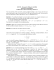### Reliable health information on ibxpress.com poster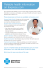### BASIC STATISTICS 1. S , R S### Setting Mitchell On-Demand v 5.8 DVD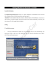### Panel Data: Fixed and Random Effects 2 Short Guides to Microeconometrics Kurt Schmidheiny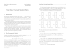### Stat 528 Lecture Notes-III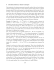### northern high school replacement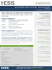### Multiplicative-error models with sample selection Discussion paper 2014-05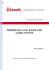### Document 6529317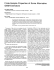### - La Gentilhommière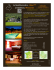### Regression Analysis of Data from a Cluster Sample### Lecture 10 - Jussi Klemelä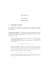### Semiparametric Estimation of a Sample Selection Model in the Presence of Endogeneity Abstract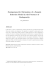### Recruiting Brochure 3-21-2011 - Kokosing Construction Company, Inc.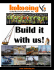### Semiparametric Estimation of a Binary Choice Model with Sample Selection Abstract J¨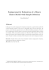### Econometrics Lecture 4: Maximum Likelihood Estimation and Large Sample Tests 1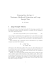### Nonparametric Estimation for Semi-Markov Processes Based on K-Sample Paths with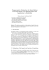### A New Estimator for Panel Data Sample Selection Models María Engracia ROCHINA-BARRACHINA*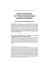### Robust Inference in Sample Selection Models Mikhail Zhelonkin , Marc G. Genton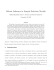### Finite sample properties of estimators of spatial autoregressive models with autoregressive disturbances